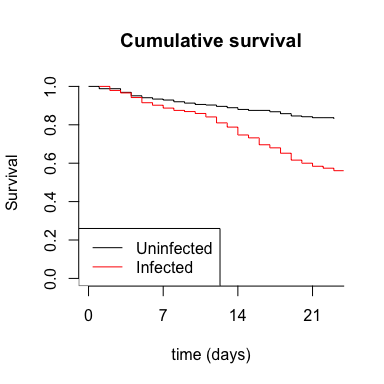# The exponential distribution

library(anovir)

### Introduction

The mathematical models describing the epidemiology of infectious diseases commonly assume mortality rates remain constant over time. This assumption is sometimes justified by observed data, particularly if they cover relatively short periods of time compared to the organism's expected survival. However assuming constant rate of mortality is more frequently a convenience, making models easier to manipulate and interpret.

The hazard function of the exponential distribution describes constant rates of mortality. This is a special case of the Weibull distribution and occurs when b = 1;

\begin{align} h(t) &= \frac{1}{bt} \exp \left( \frac{\log t - a}{b} \right) \\ \\ &= \frac{1}{t} \exp \left( \log t - a \right) \\ \\ &= \frac{1}{t} \frac{\exp \left(\log t \right)}{\exp \left(a \right)} \\ \\ &= \exp \left( -a \right) \\ \\ \end{align}

where the first line is the hazard function for the Weibull distribution with location and scale parameters a and b, respectively. When b = 1, the rate of mortality described by the hazard function is constant at, h(t) = exp(-a).

The nll_functions of this package can estimate constant rates of mortality as described by the exponential distribution. This is achieved by choosing the Weibull distribution to describe the pattern of mortality in question and constraining the scale parameter to equal one when specifying the value of variables to be sent for maximum likelihood estimation.

### Example: background rate of mortality constant

The following example uses a subset of data from Sy et al ; the full dataset is freely available at . The data record the survival of blood-fed adult female Aedes aegypti mosquitoes, either infected or not with the microsporidian parasite Vavraia culicis, over the first three weeks of the adult life. Uninfected adult Ae. aegypti females can survive much longer than this in laboratory conditions.In a first model, the Weibull distribution was chosen to describe both background mortality and mortality due to infection, d1 and d2, respectively,

    head(data_sy)
#>   sy_times sy_inf sy_censor sy_fq fq
#> 1        1      0         0     5  5
#> 2        3      0         0     8  8
#> 3        4      0         0     8  8
#> 4        5      0         0     4  4
#> 5        6      0         0     3  3
#> 6        7      0         0     2  2

m01_prep_function <- function(a1, b1, a2, b2){
nll_basic(
a1, b1, a2, b2,
data = data_sy,
time = sy_times,
censor = sy_censor,
infected_treatment = sy_inf,
d1 = 'Weibull', d2 = 'Weibull')
}

m01 <- mle2(m01_prep_function,
start = list(a1 = 3, b1 = 0.5, a2 = 3, b2 = 0.5)
)

summary(m01)
#> Maximum likelihood estimation
#>
#> Call:
#> mle2(minuslogl = m01_prep_function, start = list(a1 = 3, b1 = 0.5,
#>     a2 = 3, b2 = 0.5))
#>
#> Coefficients:
#>    Estimate Std. Error z value     Pr(z)
#> a1 4.844163   0.226726 21.3657 < 2.2e-16 ***
#> b1 1.017430   0.106821  9.5246 < 2.2e-16 ***
#> a2 3.674443   0.115807 31.7289 < 2.2e-16 ***
#> b2 0.630937   0.092334  6.8332 8.304e-12 ***
#> ---
#> Signif. codes:  0 '***' 0.001 '**' 0.01 '*' 0.05 '.' 0.1 ' ' 1
#>
#> -2 log L: 2132.742

The estimate (± s.e.) for the scale parameter, b1, overlaps with 1.0, suggesting the exponential distribution was suitable for describing background mortality.

In a second model the scale parameter for background mortality b1 was constrained, or fixed, to b1 = 1.0 throughout the estimation process. Hence background mortality was estimated according to the exponential distribution.

    m02 <- mle2(m01_prep_function,
start = list(a1 = 4.8, a2 = 3.6, b2 = 0.6),
fixed = list(b1 = 1.0)
)
summary(m02)
#> Maximum likelihood estimation
#>
#> Call:
#> mle2(minuslogl = m01_prep_function, start = list(a1 = 4.8, a2 = 3.6,
#>     b2 = 0.6), fixed = list(b1 = 1))
#>
#> Coefficients:
#>    Estimate Std. Error z value     Pr(z)
#> a1 4.813000   0.119521 40.2690 < 2.2e-16 ***
#> a2 3.680324   0.111509 33.0049 < 2.2e-16 ***
#> b2 0.636581   0.085958  7.4057 1.305e-13 ***
#> ---
#> Signif. codes:  0 '***' 0.001 '**' 0.01 '*' 0.05 '.' 0.1 ' ' 1
#>
#> -2 log L: 2132.769

# compare models by AICc
AICc(m01, m02, nobs = 753)
#>       AICc df
#> 1 2140.795  4
#> 2 2138.801  3

The log-likelihoods of the two models were very similar, indicating the data were not less well described when the exponential distribution was chosen to describe the background mortality. This was confirmed by the lower AICc of the second model, as fewer parameters were estimated for essentially the same log-likelihood indicating it was the better of the two models.